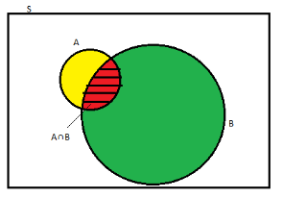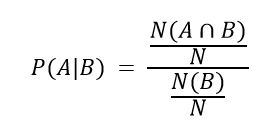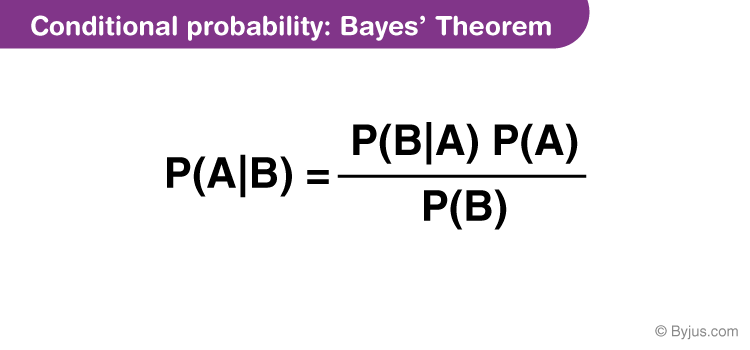# Conditional Probability

Conditional probability is known as the possibility of an event or outcome happening, based on the existence of a previous event or outcome. It is calculated by multiplying the probability of the preceding event by the renewed probability of the succeeding, or conditional, event.

Here the concept of the independent event and dependent event occurs. Imagine a student who takes leave from school twice a week, excluding Sunday. If it is known that he will be absent from school on Tuesday then what are the chances that he will also take a leave on Saturday in the same week? It is observed that in problems where the occurrence of one event affects the happening of the following event, these cases of probability are known as conditional probability.

## Definition

The probability of occurrence of any event A when another event B in relation to A has already occurred is known as conditional probability. It is depicted by P(A|B).As depicted by the above diagram, sample space is given by S, and there are two events A and B. In a situation where event B has already occurred, then our sample space S naturally gets reduced to B because now the chances of occurrence of an event will lie inside B.

As we have to figure out the chances of occurrence of event A, only a portion common to both A and B is enough to represent the probability of occurrence of A, when B has already occurred. The common portion of the events is depicted by the intersection of both the events A and B, i.e. A ∩ B.

This explains the concept of conditional probability problems, i.e. occurrence of any event when another event in relation to has already occurred.

### What is Marginal probability?

Marginal probability is the probability of an event happening, such as (p(A)), and it can be mentioned as an unconditional probability. It does not depend on the occurrence of another event. For example, the likelihood that a card is drawn from a deck of cards is black (P(black) = 0.5), and the probability that a card is drawn is 7 (P(7)=1/13), both are independent events since the outcome of another event does not condition the result of one event.

### What is Joint Probability?

A joint probability is the probability of event A and event B happening, P(A and B). It is the likelihood of the intersection of two or more events. The probability of the intersection of A and B is written as P(A ∩ B). For example, the likelihood that a card is black and seven is equal to P(Black and Seven) = 2/52 = 1/26. (There are two Black-7 in a deck of 52: the 7 of clubs and the 4 of spades).

## Formula

When the intersection of two events happen, then the formula for conditional probability for the occurrence of two events is given by;

 P(A|B) = N(A∩B)/N(B) Or P(B|A) = N(A∩B)/N(A)

Where P(A|B) represents the probability of occurrence of A given B has occurred.

N(A ∩ B) is the number of elements common to both A and B.

N(B) is the number of elements in B, and it cannot be equal to zero.

Let N represent the total number of elements in the sample space.Since N(A ∩ B)/N and N(B)/N denotes the ratio of the number of favourable outcomes to the total number of outcomes; therefore, it indicates the probability.

Therefore, N(A ∩ B)/N can be written as P(A ∩ B) and N(B)/N as P(B).

P(A|B) = P(A ∩ B)/P(B)

Therefore, P(A ∩ B) = P(B) P(A|B) if P(B) ≠ 0

= P(A) P(B|A) if P(A) ≠ 0

Similarly, the probability of occurrence of B when A has already occurred is given by,

P(B|A) = P(B ∩ A)/P(A)

To have a better insight, let us practice some conditional probability examples.

### Conditional Probability and Bayes Theorem

Bayes’ theorem defines the probability of occurrence of an event associated with any condition. It is considered for the case of conditional probability. Also, this is known as the formula for the likelihood of “causes”.A tree diagram will help you in understanding the probability of different events in different cases.

## Properties

Property 1: Let E and F be events of a sample space S of an experiment, then we have:

P(S|F) = P(F|F) = 1.

Property 2: If A and B are any two events of a sample space S and F is an event of S such that P(F) ≠ 0, then;

P((A ∪ B)|F) = P(A|F) + P(B|F) – P((A ∩ B)|F)

Property 3: P(A′|B) = 1 − P(A|B)

## Problems and Solutions

Example 1: Two dies are thrown simultaneously, and the sum of the numbers obtained is found to be 7. What is the probability that the number 3 has appeared at least once?

Solution: The sample space S would consist of all the numbers possible by the combination of two dies. Therefore S consists of 6 × 6, i.e. 36 events.

Event A indicates the combination in which 3 has appeared at least once.

Event B indicates the combination of the numbers which sum up to 7.

A = {(3, 1), (3, 2), (3, 3)(3, 4)(3, 5)(3, 6)(1, 3)(2, 3)(4, 3)(5, 3)(6, 3)}

B = {(1, 6)(2, 5)(3, 4)(4, 3)(5, 2)(6, 1)}

P(A) = 11/36

P(B) = 6/36

A ∩ B = 2

P(A ∩ B) = 2/36

Applying the conditional probability formula we get,

P(A|B) = P(A∩B)/P(B) = (2/36)/(6/36) = ⅓

Example 2: In a group of 100 computer buyers, 40 bought CPU, 30 purchased monitor, and 20 purchased CPU and monitors. If a computer buyer chose at random and bought a CPU, what is the probability they also bought a Monitor?

Solution: As per the first event, 40 out of 100 bought CPU,

So, P(A) = 40% or 0.4

Now, according to the question, 20 buyers purchased both CPU and monitors. So, this is the intersection of the happening of two events. Hence,

P(A∩B) = 20% or 0.2

By the formula of conditional probability we know;

P(B|A) = P(A∩B)/P(B)

P(B|A) = 0.2/0.4 = 2/4 = ½ = 0.5

The probability that a buyer bought a monitor, given that they purchased a CPU, is 50%.

## Frequently Asked Questions – FAQs

### How do you find conditional probability?

Suppose A and B be the two independent events with probabilities respectively P(A) and P(B) such that the probability of occurrence of event B given A is given by:
P(B|A) = P(A ∩ B)/P(A)

### What is the difference between probability and conditional probability?

The main difference between the probability and the conditional probability is that probability is the likelihood of occurrence of an event say A, whereas the conditional probability defines the probability of an event by assuming another event has already occurred, i.e. in the conditional probability of A given B, the event B is assumed to have already occurred.

### Why do we need conditional probability?

Conditional probability is required when some events may occur in relation to the occurrence of another event.

### What does given mean in probability?

While writing the conditional probability of A given B, i.e. P(A|B), the symbol “|” represent the word “given”. This means the event after “|” is already occurred.

### What is an example of conditional probability?

Suppose three cards from a deck of 52 cards are missing. Now the probability of picking up a card from this deck is a diamond can be found using the conditional probability.

Test your knowledge on Conditional Probability